# Online Mechanical Engineering Test - Strength of Materials Test 2Loading Test...

Instruction:

• This is a FREE online test. DO NOT pay money to anyone to attend this test.
• Total number of questions : 20.
• Time alloted : 30 minutes.
• Each question carry 1 mark, no negative marks.
• DO NOT refresh the page.
• All the best :-).

1.

Strain energy is the

A.
 energy stored in a body when strained within elastic limitsB.
 energy stored in a body when strained upto the breaking of a specimenC.
 maximum strain energy which can be stored in a bodyD.
 proof resilience per unit volume of a material2.

The stress induced in a body, when suddenly loaded, is __________ the stress induced when the same load is applied gradually.

A.
 equal toB.
 one-halfC.
 twiceD.
 four times3.

When a rectangular beam is loaded transversely, the maximum compressive stress is developed on the

A.
 top layerB.
 bottom layerC.
 neutral axisD.
 every cross-section4.

Resilience is the

A.
 energy stored in a body when strained within elastic limitsB.
 energy stored in a body when strained upto the breaking of the specimenC.
 maximum strain energy which can be stored in a bodyD.
 none of the above5.

If the tearing efficiency of a riveted joint is 50%, then ratio of rivet hole diameter to the pitch of rivets is

A.
 0.2B.
 0.3C.
 0.5D.
 0.66.

The stress at which the extension of the material takes place more quickly as compared to the increase in load, is called

A.
 elastic limitB.
 yield pointC.
 ultimate pointD.
 breaking point7.

The bending moment in the centre of a simply supported beam carrying a uniformly distributed load of w per unit length is

A.
 zeroB.
 wl2/2C.
 wl2/4D.
 wl2/88.

When a closely-coiled helical spring is subjected to an axial load, it is said to be under

A.
 bendingB.
 shearC.
 torsionD.
 crushing9.

The given figure shows the Mohr's circle of stress for two unequal and like principal stresses (σx and σy) acting at a body across two mutually perpendicular planes. The normal stress on an oblique section making an angle θ with the minor principle plane is given by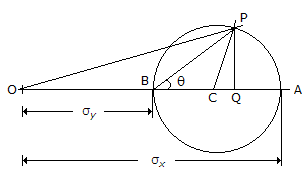A.
 OCB.
 OPC.
 OQD.
 PQ10.

The unit of modulus of elasticity is same as those of

A.
 stress, strain and pressureB.
 stress, force and modulus of rigidityC.
 strain, force and pressureD.
 stress, pressure and modulus of rigidity11.

The horizontal thrust offered by the retaining wall on the retained material is (where w = Specific weight of the retained material, h = height of retaining wall, and (φ) = Angle of repose of the retained earth)

A.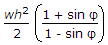B.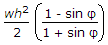C.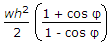D.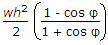12.

The bending moment at a section tends to bend or deflect the beam and the internal stresses resist its bending. The resistance offered by the internal stresses, to the bending, is called

A.
 compressive stressB.
 shear stressC.
 bending stressD.
 elastic modulus13.

For the two shafts connected in parallel and subjected to twisting moment, the angle of twist of each shaft will be same.

A.
 YesB.
 No14.

Poisson's ratio is the ratio of linear stein to the volumetric strain.

A.
 TrueB.
 False15.

The bending moment at the free end of a cantilever beam is

A.
 zeroB.
 minimumC.
 maximum16.

The lower layer of the beam as shown in the below figure, will be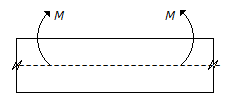A.
 in tensionB.
 in compressionC.
 neither in tension nor in compression17.

If percentage elongation of a certain specimen made of a material 'A' under tensile test is 30% and the percentage elongation of a specimen with same dimensions made of another material 'B' is 40%, then material 'B' is more ductile than material 'A'.

A.
 CorrectB.
 Incorrect18.

The section modulus of a circular section about an axis through its C.G., is

A.
 πd2/4B.
 πd2/16C.
 πd3/16D.
 πd3/3219.

The length of a conical bar is l, diameter of base is d and weight per unit volume is w. It is fixea at its upper end and hanging freely. The elongation of the bar under the action of its own weight will be

A.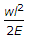B.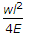C.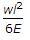D.20.

The Rankine's constant for a mild steel column with both ends hinged is

A.
 1/750B.
 1/1600C.
 1/7500D.
 1/9000